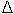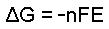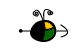Non-Standard State Free Energy ChangesG, the free energy change of a chemical process under non-standard state conditions (concentrations not 1 molar and pressures not 1 atmosphere) can be determined two different ways:

• From the Standard State Free Energy Change and the Reaction Quotient
• From Cell Potentials
• Using a Standard State Free Energy Change and the Reaction Quotient to Determine a Non-standard State Free Energy Change

If we know the standard free energy change for a process,Go, and the reaction quotient, Q, for the change we can determine the non-standard state free energy change,G, for the process using the following equation:In this equation:

• R = 8.314 J mol-1 K-1 or 0.008314 kJ/mol-1 K-1.
• T is the temperature on the Kelvin scale.
• ln Q is the natural logarithm of the reaction quotient.

Top

Using Cell Potentials to Determine Non-standard State Free Energy Changes

If we know the potential, E, for an electrochemical cell operating at non-standard state conditions (or if we can determine the cell potential) we can calculate the free energy change,G, for the cell reaction using the equation:In this equation:

• n is the number of moles of electrons exchanged in the cell reaction.
• F is 96.485 kJ volt-1 mol-1 (the "Faraday").
• E is the cell potential under non-standard state conditions (concentrations not 1 molar, pressures not 1 atmosphere).

Top